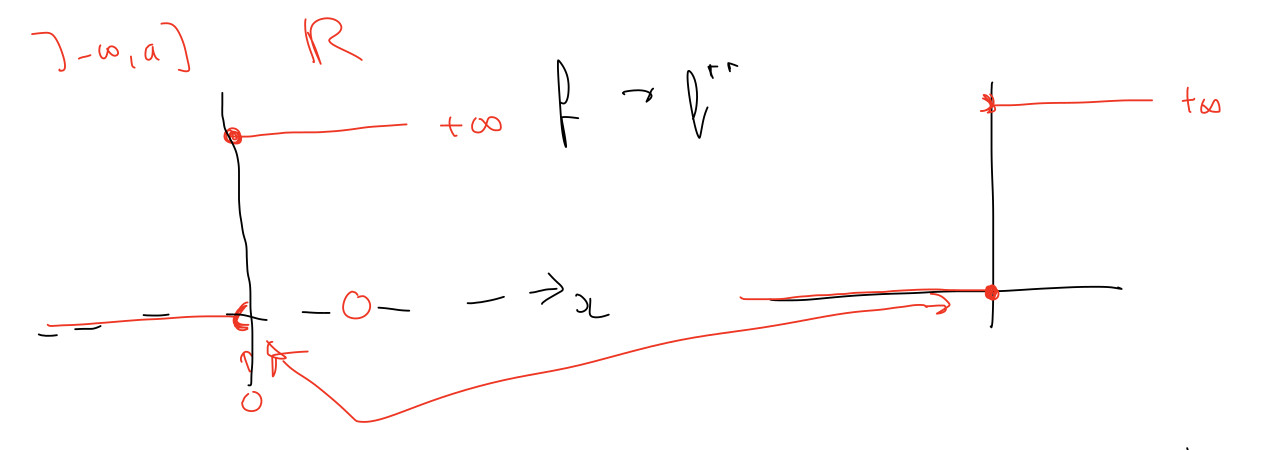### Exercise 10, handnotes, open function example

Hi,

On the third page of hand notes of exercise 10, there is an example of an open convex function $$f(x)$$ and its conjugate of conjugate. I'm wondering how the conjugate of $$f$$ is computed for $$y>0$$ in this case.

I see that $$x^Ty$$ is always below $$f(x)$$ for positive $$y$$, and never intersects with $$f(x)$$, so the gap is always negative. But for values of $$x$$ slightly smaller than zero, $$x^Ty - f(x)$$ is very close but to equal to zero. So it's not clear to me how $$f^*(y)$$ is computed here.Top comment

Hello,

Contrary to the lecture notes, the handwritten notes use 'sup' instead of 'max'. This is exactly to take care of the issue you raise. Indeed, for $$f(x)=0 \text{ if } x < a$$, if $$u > 0$$, $$\max_x u x - f(x)$$ is something like $$u(a - \epsilon)$$ for any small positive $$\epsilon$$. 'sup' resolves this limit, so the conjugate$$f(x)$$ value would be $$ua$$.

Does that help?

Hello,

Where do these handwritten notes come from ? I never saw them and cannot find them on github.